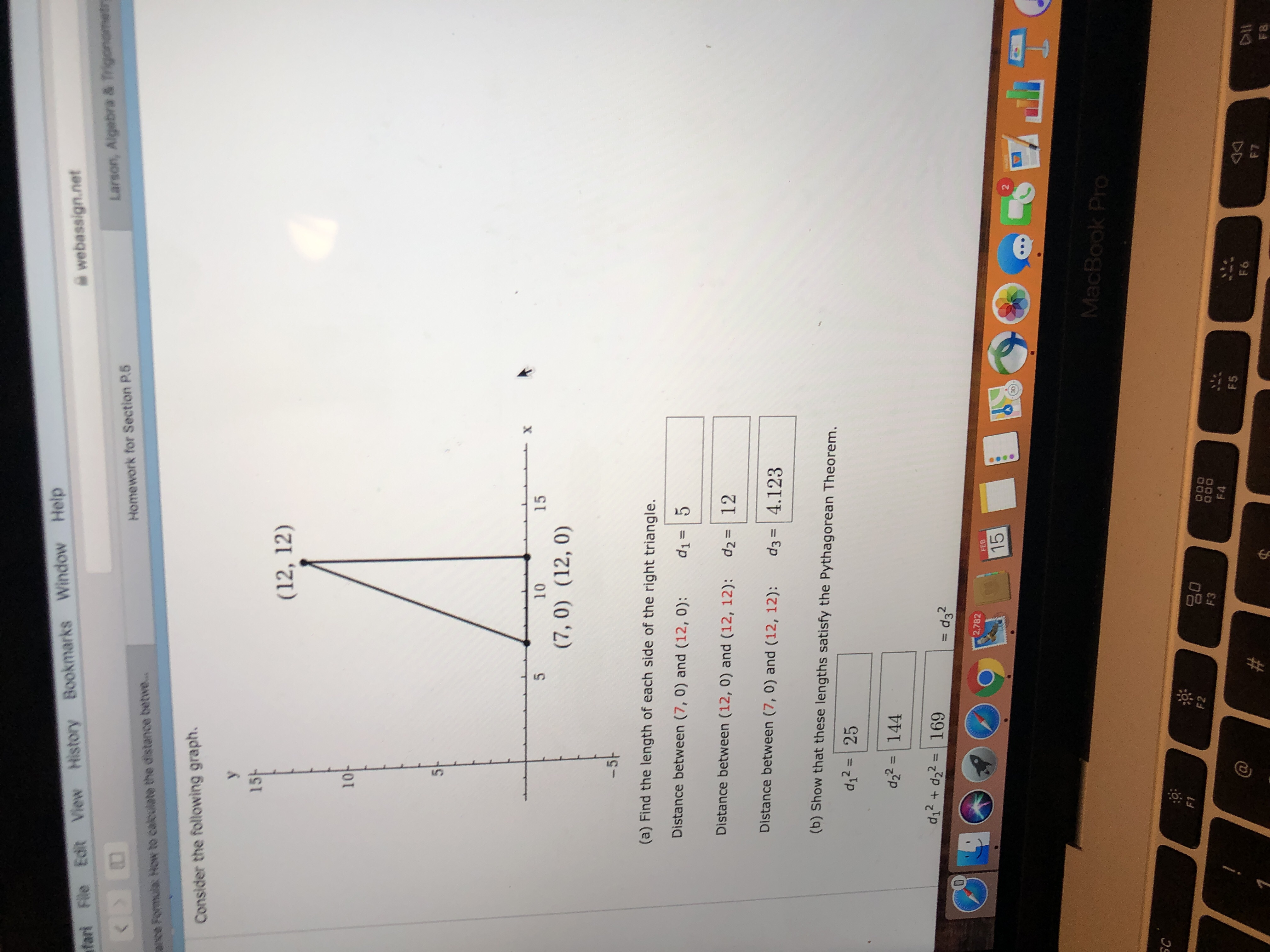# File Edit View History Bookmarks Window Help " webassign.net fari Larson, Algebra & Homework for Section P6 ance Formula How to caloulete the distance betwe Consider the following graph. 바 15 (12, 12) 10 10 15 (7, 0) (12, 0) (a) Find the length of each side of the right triangle Distance between (7, 0) and (12,0): d Distance between (12, 0) and (12, 12): d2 12 Distance between (7, 0) and (12, 12): d34.123 5 (b) Show that these lengths satisfy the Pythagorean Theorem d2 25 df= | 144 d12 d22169 2 3 2,782 FED 15 SC F3 F4 F5 F7 F 8

Question

I need help with my homework. I just need someone to confirm if I’m doing it righthelp_outlineImage TranscriptioncloseFile Edit View History Bookmarks Window Help " webassign.net fari Larson, Algebra & Homework for Section P6 ance Formula How to caloulete the distance betwe Consider the following graph. 바 15 (12, 12) 10 10 15 (7, 0) (12, 0) (a) Find the length of each side of the right triangle Distance between (7, 0) and (12,0): d Distance between (12, 0) and (12, 12): d2 12 Distance between (7, 0) and (12, 12): d34.123 5 (b) Show that these lengths satisfy the Pythagorean Theorem d2 25 df= | 144 d12 d22169 2 3 2,782 FED 15 SC F3 F4 F5 F7 F 8 fullscreen

### Want to see this answer and more?

Experts are waiting 24/7 to provide step-by-step solutions in as fast as 30 minutes!*

*Response times vary by subject and question complexity. Median response time is 34 minutes and may be longer for new subjects.
Tagged in
MathCalculus

### Other